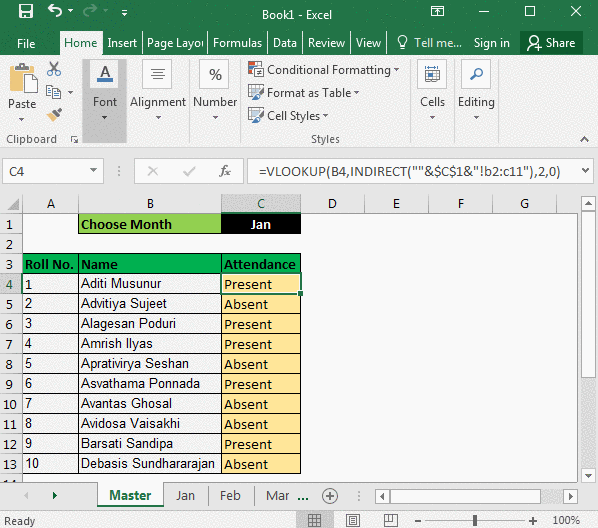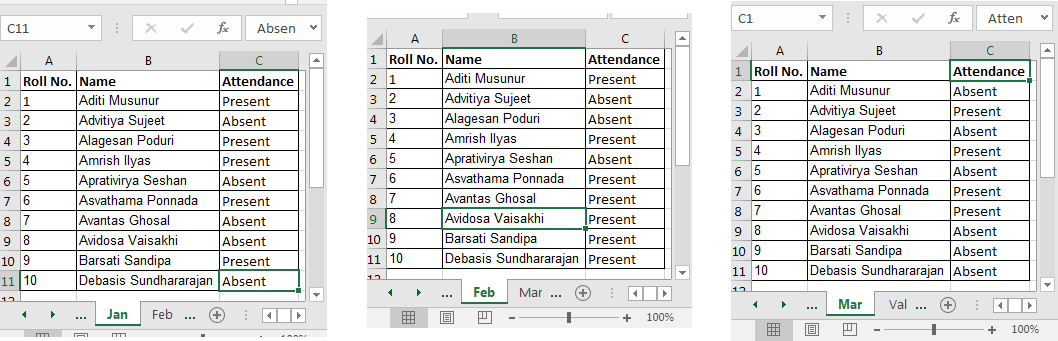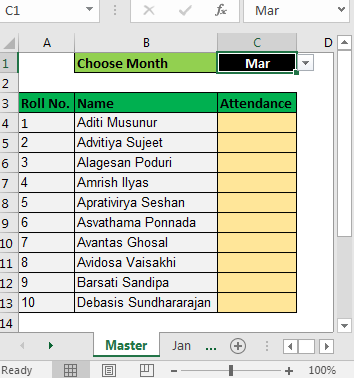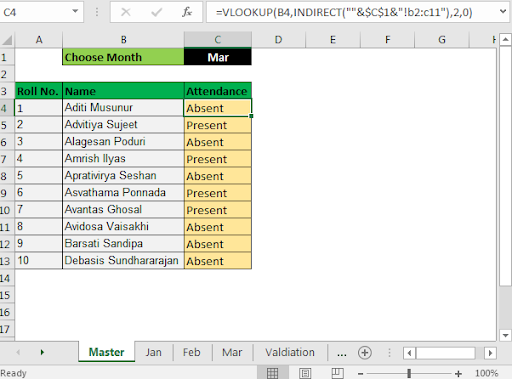Select From Drop Down And Pull Data From Different Sheet in Microsoft Excel 2016Let's say you are a teacher. You have prepared attendance of students in a workbook. Each month’s attendance is in a different sheet of that month’s name.

In a master sheet, you want to put a VLOOKUP to check if that student was present or absent that month. It would be simple if your data was on the same sheet, but it is not. They are on different sheets. But it doesn’t mean we can’t pull data from another sheet in excel. We can and will.

For the month, you have created a drop-down in cell C1. It contains a list of months. Now you want to show absent or present based on the selected month in Cell C1. Let's just see the generic formula first.

Generic Formula for VLOOKUP from Multiple Sheets:

 =VLOOKUP(lookupValue,INDIRECT(""&cell that contains name of month&"!range"),col_index_no,0)

For this example, I have my attendance in “Jan”, “Feb”, and “Mar” sheets in the same range A2:C11.Now I have prepared a master sheet.In cell C4, put this formula and drag it down.

 =VLOOKUP(B4,INDIRECT(""&\$C\$1&"!B2:C11"),2,0)Now, whenever you change the month’s name in cell C1, excel will pull value from another sheet (from that month's sheet, if it exists).

Explanation:

We used  Excel Indirect function to fetch value from another sheet.

INDIRECT Function changes the text into reference.We used INDIRECT for referencing other sheets in excel.

For example, if you write INDIRECT(“sheet2:A2”) in a1 on sheet1. It will pull the value from sheet2!A2 in sheet1:A1. If you write =VLOOKUP(“abc”,INDIRECT(“sheet2!A2:B100”),2,0)  any sheet,
VLOOKUP will look for “abc” in range A2:B100 on sheet2.

INDIRECT(""&\$C\$1&"!B2:C11") : Here we want sheet name to change, that is why we have written it like this. If Cell C1 contains “Jan”, it will translate to INDIRECT(“Jan!B2:C11”) which will then translate to Jan!B2:C11 for VLOOKUP table array. If C1 has Feb it will translate to INDIRECT(“Feb!B2:C11”) and so on.

Afterwards, VLOOKUP did his job.

VLOOKUP(B4,INDIRECT(""&\$C\$1&"!B2:C11"),2,0): now since Indirect gave the table array, VLOOKUP simply pulls data from that range easily.

So we learned how to reference different sheets using Indirect. How to pull data from different sheets in excel using VLOOKUP and INDIRECT functions. All these functions are available in all excel versions, including Excel 2016, Excel 2013 and Excel 2010.

Related Articles:

How to VLOOKUP from Different Excel Sheet

Work With Named Ranges on Different Sheets in Excel

How to Get All Matches in Different Columns

Popular Articles:

50 Excel Shortcut to Increase Your Productivity

The VLOOKUP Function in Excel

COUNTIF in Excel 2016

How to Use SUMIF Function in Excel

1.